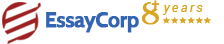WhatsApp
Struggling With Assignments?

We are here to help.

Chat with our expert writers on WhatsApp

for any kind of assignment queries.

Click to chat!
Struggling with Assignments? WhatsApp Us## Introduction

This report discusses the design and analysis of a two stage amplifier. An FET based common source amplifier was designed.FET was preferred over BJT because of the following reasons.

• FETs are voltage operated devices, so consumes less power.
• FETs have high input impedance

Common source amplifier was preferred over Common gate or common drain because of its high gain, high bandwidth and high input impedance.

## Specifications

of the Amplifier

Number of stages: 2

Bandwidth: 10 KHz Gain: 2.8

## Circuit Diagram

Two identical single stage amplifiers are combined to get a two-stage amplifier.Fig: 1 shows the circuit diagram of the common source two stage amplifier

R1 and R2 are used for biasing the MOSFET. They determine the input impedance of the amplifier. They must be high to have a high gain.C1 is the input decupling capacitor, which is used to remove the DC component of the signal to be amplified. Its value must be high to have a high gain at lower signal frequency. It determines low frequency gain of the amplifier. RD determines the gain of the amplifier. For higher gain RD must be high.C2 is called bypass capacitor, used to bypass the signal to ground. When C2 is removed, signal will get dropped across RS and it gives a negative feedback. So gain reduces. If C2 is not present and neglecting the effect of C1 gain will be gm R1/(1+ gmR2).

#### DC Analysis

For DC analysis all the capacitors are neglected ( considered as open circuit).

Since both the stages are identical, analysis of only signle stage is presented here.

VDD= 10V

VDS = VDD/2 = 5V

Take ID= 0.1 mA

ID = (VDD -VDS)/(RD+RS) = 5/(RD+RS)

RD + RS = 5/0.1 mA = 50 K;

Take RD = RS = 25K

Gate Current, IG = 0 mA

Let R1 = R2 = R

VG= VDD/2 = 5V

Input impedance is determined by R1 and R2. In order to avoid loading of the signal source, we need to select a high value of R1 and R2.

Take R1 = R2 = 1M

HIRE EXPERT
Email ID *
Subject
Pages